# Construction Calculators (Bric

100K+Everyone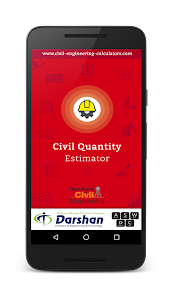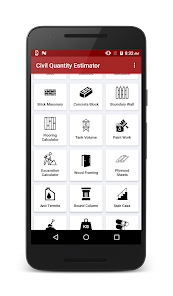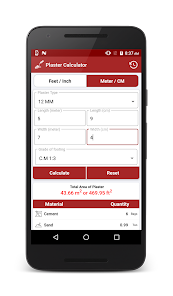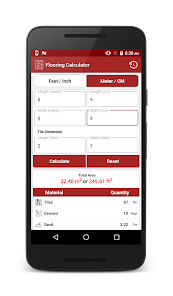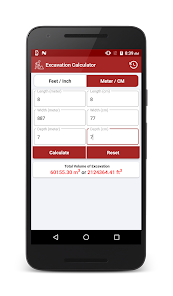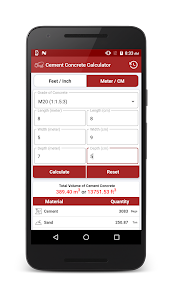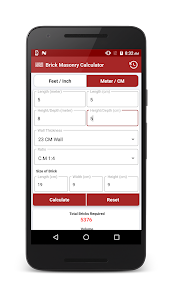Civil Quantity Estimator contains the set of calculators for the estimation of Cement Concrete, Clay Bricks, Cement Blocks, Paint, Steel, Flooring, Compound wall, Plastering, Tank Volume, Excavation, etc.
Construction / House cost & material quantity Estimator
It helps to finalize the approximate amount of cost & quantity of material required for constructing a home. It estimates the approx cost and quantity of Cement, Sand, Aggregate, Steel, Paint, Flooring, Tiles, Bricks, Window, Doors, Plumbing, Electrical, etc.
Brick Masonry / Clay Brick Calculator

It calculates the number of bricks and mortar required for a given wall area. Based on wall length, wall height/depth, wall thickness, Brick Size & Cement concrete Ratio, it calculates the number of bricks required, quantity of cement required in Bags & quantity of sand required in Ton
Concrete/Solid Block Calculator
It calculates the number of concrete solid blocks and mortar required for a given wall area. Based on wall length, wall height/depth, wall thickness, Concrete block Size & Cement concrete Ratio, it calculates the number of concrete solid blocks required, the quantity of cement required in Bags & the quantity of sand required in Ton.
Cement Concrete/PCC/RCC Calculator
It calculates the number of cement bags, amount of sand required in Ton & amount of Aggregate required in Ton for a total volume of Cement Concrete based on Grade of Concrete (M20, M15, M10, M7.5), length/width/depth of the construction
Plastering Calculator
Calculate the number of cement bags and sand required for wall plastering using a plastering calculator. Users can also calculate the amount of cement required for the ceiling, external rough walls also.
Precast Boundary / Wall fencing calculator
It is used to calculate the quantity of precast member (precast panel / precast slab) & precast post required for given length and height of compound boundary.
Flooring (Tiles) Calculator
Calculate the number of tiles, amount of cement and sand for a given flooring area.
Tank capacity calculator
It calculates water tank capacity in liters, Calculate capacity and fill volumes of common tank shapes for water, oil, or other liquids.
Paint/color Calculator
It helps to calculate the area to be painted and gives an estimate of the required amount of paint, primer & putty.
Excavation calculator
It helps to calculate amount of land/earth needs to be excavated for a house or building construction. User can calculate the length, breadth, and depth of the site for excavation.
Plywood sheets calculator
It estimates the required number of plywood pieces needed based on Room area.
Anti Termite calculator
It calculates the amount of anti-termite chemical required for any construction.
Topsoil Calculator
It calculates the quantity of topsoil needed to cover the area of the garden or yard.
Staircase/ Stair Steps Calculator
It determines stair parameters such as rise, total treads, angle, and stringer length, based on height, run and tread requirement. It calculates the volume of the staircase & the amount of cement, sand, and aggregates required for the construction of the staircase.
Carpet Area, Built-Up Area, Super Built-Up area Calculator
It calculates carpet area, Built-up area, and super built-up area for a given Plan.
Wood Framing
It calculates the volume of wood/lumber in cubic feet (Cft)
Concrete Round Column Calculator
It is used to determine how much cement bags, sand, and aggregate are required for the preparation of the given dimension Round Column.
Steel weight calculator
It calculates an estimate of the weight of different types of Steel materials based on dimensions.
Concrete Pipe Calculator
It is used to calculate the volume of pipe & estimate the number of cement bags, sand & aggregates require to make the concrete pipe for water drainage, sewerage, & irrigation.
Updated on
Aug 22, 2021

## Data safety